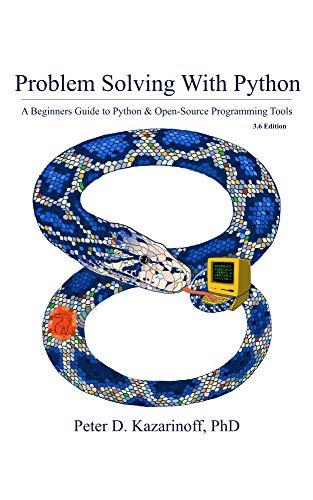## Plotting a Gaussian normal curve with Python and MatplotlibIn the previous post, we calculated the area under the standard normal curve using Python and the erf() function from the math module in Python's Standard Library. In this post, we will construct a plot that illustrates the standard normal curve and the area we calculated. To build the Gaussian …

## Calculating the probability under a normal curve with PythonCalculating the probability under a normal curve is useful for engineers. This type of calculation can be helpful to predict the likely hood of a part coming off an assembly line being within a given specification. The probability can be calculated when the statistical properties of all the parts that …

## My book Problem Solving with Python is available on AmazonMy first book: Problem Solving with Python 3.6 Edition is available on Amazon. So excited about publishing my first book! Pick it up here: Link to Amazon

The book has been over a year in the making. Many long nights writing and revising. Without the support of my awesome …

## Using Python to control an ArduinoPython is used in many applications including data science, machine learning, and web development. Another area where we can use Python is external hardware control. What do I mean by external hardware? A piece of external hardware could be a light or a sensor. External hardware includes multimeters or spectral …

## A List of Python Learning ResourcesThis post is a short list of my recommended Python learning resources. I received an email from someone who found this blog and my GitHub repo and asked about the right place to go to start learning Python. The list of resources in this post comes from the email I …